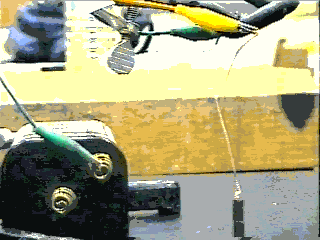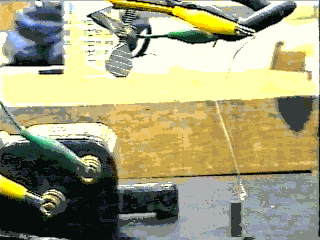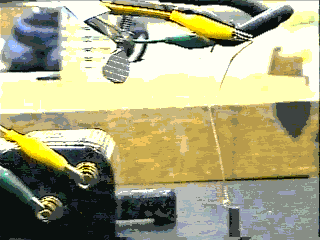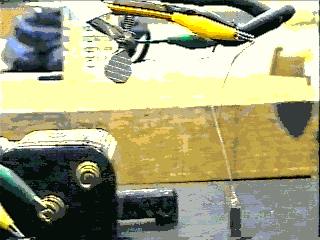Lorentz ElectroMagnetic DEFLECTION: action-at-a-distance (part 1)

The Lorentz Force Law assumes that, if you already have a wire conducting a significant DC current, and it is positioned in the presence of another magnetic field, then your wire will be deflected by a magnetic force. The direction of this deflection force depends only on the direction of the current in your wire as well as the direction of the magnetic field you place this wire in. If you either reverse the direction of the DC current you use, or reverse the polarity of the magnetic field you are using, then you reverse the direction of the deflection force. Of course, if you position your wire (along with its DC current) too far from the magnetic field, you may notice a very weak deflection or none at all; no matter how much DC current you use. You may even melt your wire instead, if you use too much DC current.

Magnitude ("strength") of the "Lorentz Deflection":

F = ILB

This is just a special case of the more general form:

F = qE + qvB

Here:

E = External electric field = exactly zero (or so small that it cannot be measured).

B = External magnetic field, which weakens by factor (1/r) as r increases, where r = distance (or closest radius) the exposed wire is from the pole's tip on a toy permanent magnet. However, inside a solenoid, or between two solenoid ends (or dipole sides) that are sufficiently close together, B is uniform and constant with respect to distance r from the common axis of all wire "windings" or "loops" involved. Under these conditions, if radius r exceeds that of the involved wire loops or windings, B is negligibly small or non-existent. Please refer to the comment below about our "crude, round-about" assumption of our B-field.

"B inside a solenoid" and sufficiently away from its ends toward its half-length:

B = (pi)(.0000004)(current I)(total loop count) / (length of solenoid). This is where "B" for solenoids is the strongest, most uniform and constant, regardless of value for "r", unless you go too close the solenoid's ends (gets weaker and less uniform and not so constant) or outside the solenoid's windings/loops (it practically vanishes out here!).

"B at the center of a dipole": B = (.0000002)(total loop count)(current I)(circular area) / (r cubed).

"B around any single wire", as a result of its own current conducted: B = (.0000002)(current I) / r.

q = electric charge, and v = velocity of that electric charge q.

We can (safely) allow electric current "I" to represent our "moving charge" in question, that is:

I= qv.

L = Total Length of exposed or immersed wire at 90 degrees to the magnetic field's "lines of force" (and "immersed" in this field, if we can draw such imaginary rubber-band-like lines from magnetic N-pole to S-pole, they indicate the field's "direction", "position", and "strength".)

For the sake of your own convenience, we can make this "crude, round-about" empirical measurement:

The following assumption is not to be misinterpreted as "concrete" and "sound" theory, but rather it is provided to allow you a physical appreciation of the Lorentz Deflection. That is, "Don't just take my word for it, but try this out yourself and verify it by FINDING OUT FOR YOURSELF".

Let B = P/r, where we allow P = "pole's strength" = Fr/(IL), where you measure (as best you can in S.I. "mks" metric units) quantities F, r, L, and I. This will help you to use F = ILP/r to predict future resulting Deflections. By knowing P already as well as values of any three other variables, one can now predict the fourth variable's value.

The resulting force F must be in "Newtons"; shortest distance r in meters, length of exposed wire in meters; and electric current I in "Amperes" using an ammeter (SHORTCUT: measure the voltage you applied in Volts, via voltmeter; and divide this by the electrical resistance of your circuit in Ohms, via ohmmeter). You must be sure about how to use these devices properly, otherwise they are easily damaged.

Metric unit conversion:

To experience 1 Newton of force, pick up and hold roughly 100g against the earth's own gravitation.

Remember that 1 Kg (= 1000g ("grams")) is about 2.2 lbs., in English standard weight.

Also, 100cm = 1m ("meter").

Direction of the "Lorentz Deflection":

Using your RIGHT hand, try the following:

1. Point your RIGHT INDEX finger in the direction of the DC current you are using.
2. Holding your RIGHT MIDDLE finger exactly at 90 degrees to your right index finger, point it in the direction of the magnetic field, pointing from N pole to S pole.
3. While holding your RIGHT THUMB exactly 90 degrees to BOTH your right index and right middle fingers, your RIGHT THUMB points the direction of the Lorentz Deflection.

What actually happens to produce this deflection is that the DC current you apply creates its own magnetic field around the thickness of the wire. If you point your RIGHT THUMB in the direction of the DC current used, your RIGHT FINGERS will point the direction of the wire's own magnetic field. Like magnetic poles tend to repel, and that is what produces the Lorentz Deflection.BACK TO PREVIOUS PAGE.

ONWARD WITH THIS DEMONSTRATION.